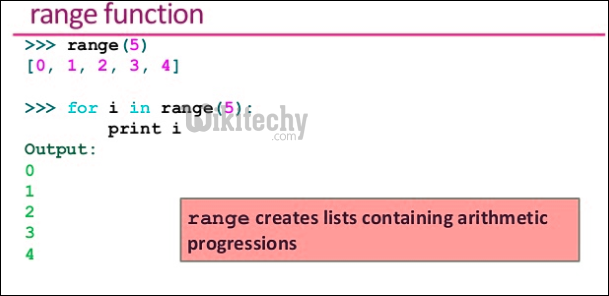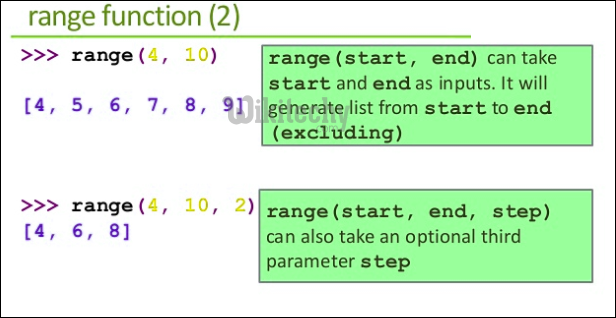# python tutorial - Python range | range() vs xrange() in Python - learn python - python programmingLearn Python - Python tutorial - python ranges - Python examples - Python programs

range() and xrange() are two functions that could be used to iterate a certain number of times in for loops in Python. In Python 3, there is no xrange , but the range function behaves like xrange in Python 2.If you want to write code that will run on both Python 2 and Python 3, you should use range().range() – This returns a list of numbers created using range() function.
xrange() – This function returns the generator object that can be used to display numbers only by looping. Only particular range is displayed on demand and hence called “lazy evaluation“.Both are implemented in different ways and have different characteristics associated with them. The points of comparisons are:

• Return Type
• Memory
• Operation Usage
• Speed
Return Type

range() returns – the list as return type.
xrange() returns – xrange() object.

python - Sample - python code :

python tutorial - Output :

```The return type of range() is :
<type 'list'="">
The return type of xrange() is :
<type 'xrange'="">
</type></type>```

Memory

The variable storing the range created by range() takes more memory as compared to variable storing the range using xrange(). The basic reason for this is the return type of range() is list and xrange() is xrange() object.

python - Sample - python code :

python tutorial - Output :

```The size allotted using range() is :
80064
The size allotted using xrange() is :
40
```
Operations usage

As range() returns the list, all the operations that can be applied on the list can be used on it. On the other hand, as xrange() returns the xrange object, operations associated to list cannot be applied on them, hence a disadvantage.

python - Sample - python code :

Error:

```Traceback (most recent call last):
File "1f2d94c59aea6aed795b05a19e44474d.py", line 18, in
print (x[2:5])
TypeError: sequence index must be integer, not 'slice'
```

python tutorial - Output :

```The list after slicing using range is :
[3, 4, 5]
The list after slicing using xrange is :
```

Speed

Because of the fact that xrange() evaluates only the generator object containing only the values that are required by lazy evaluation, therefore is faster in implementation than range().

Important Points:

• If you want to write code that will run on both Python 2 and Python 3, use range() as the xrange funtion is deprecated in Python 3
• range() is faster if iterating over the same sequence multiple times.
• xrange() has to reconstruct the integer object every time, but range() will have real integer objects. (It will always perform worse in terms of memory however)

Wikitechy tutorial site provides you all the learn python , python programming online training , python course online , python programming course